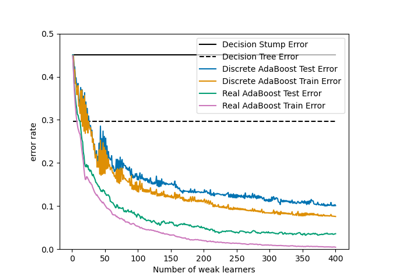# sklearn.metrics.zero_one_loss¶

sklearn.metrics.zero_one_loss(y_true, y_pred, *, normalize=True, sample_weight=None)[source]

Zero-one classification loss.

If normalize is True, return the fraction of misclassifications (float), else it returns the number of misclassifications (int). The best performance is 0.

Read more in the User Guide.

Parameters:
y_true1d array-like, or label indicator array / sparse matrix

Ground truth (correct) labels.

y_pred1d array-like, or label indicator array / sparse matrix

Predicted labels, as returned by a classifier.

normalizebool, default=True

If False, return the number of misclassifications. Otherwise, return the fraction of misclassifications.

sample_weightarray-like of shape (n_samples,), default=None

Sample weights.

Returns:
lossfloat or int,

If normalize == True, return the fraction of misclassifications (float), else it returns the number of misclassifications (int).

accuracy_score

Compute the accuracy score. By default, the function will return the fraction of correct predictions divided by the total number of predictions.

hamming_loss

Compute the average Hamming loss or Hamming distance between two sets of samples.

jaccard_score

Compute the Jaccard similarity coefficient score.

Notes

In multilabel classification, the zero_one_loss function corresponds to the subset zero-one loss: for each sample, the entire set of labels must be correctly predicted, otherwise the loss for that sample is equal to one.

Examples

>>> from sklearn.metrics import zero_one_loss
>>> y_pred = [1, 2, 3, 4]
>>> y_true = [2, 2, 3, 4]
>>> zero_one_loss(y_true, y_pred)
0.25
>>> zero_one_loss(y_true, y_pred, normalize=False)
1


In the multilabel case with binary label indicators:

>>> import numpy as np
>>> zero_one_loss(np.array([[0, 1], [1, 1]]), np.ones((2, 2)))
0.5


## Examples using sklearn.metrics.zero_one_loss¶